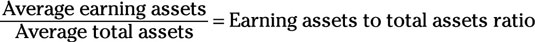##### Corporate Finance For DummiesBank analysts want to know what percentage of a company's assets are actually generating income. They determine this with the earning assets to total assets ratio. Of all the assets that a company owns (referred to as total assets), analysts want to know what percentage of them are actually generating income.

Earning assets usually include any assets that are directly generating income, such as interest-generating investments or income-generating rentals, but in some cases, they include other forms of assets that directly contribute to income, such as machinery, computers, or anything that is directly involved in producing goods and services that will be sold to customers.

You calculate the earning assets to total assets ratio by using this equation:Follow these steps to put this equation to use:

1. Use the balance sheets from the current year and previous year to find the average earnings assets and the average total assets:

• Add the earning assets from the current year and previous year and divide the answer by 2; this is the average earning assets.

• Add the total assets from the current year and previous year and divide the answer by 2; this is the average total assets.

2. Divide the average earning assets by the average total assets to get the earning assets to total assets ratio.

For companies that generate their income from loans and rentals, such as banks, a high ratio indicates a very efficient use of assets. A low ratio may indicate a poor use of assets and a need to either decrease their asset costs or improve volume.

For all other companies, analysts can use this ratio to determine how effectively the companies are generating earnings with their underutilized assets. Companies in any industry can also include any assets that are directly involved in the production of their products as an earning asset to evaluate their asset management.